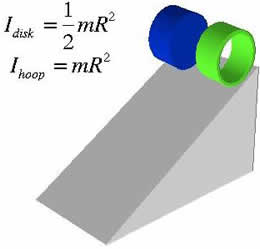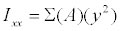# Difference between Inertia and Moment of Inertia

##### Key Difference: Inertia can be described as a property or tendency of an object that resists any change to its state of motion. Moment of Inertia is the measurement of an object’s resistance to change its rotation.Inertia can be described as a property or tendency of an object that resists any change to its state of motion. Thus, a body stays at rest or continues its motion, unless acted on by an external force. The Latin root for inertia is the same root for "inert," which refers to the lacking ability to move. Galileo, a scientist of the seventeenth century developed the concept of inertia by stating that a moving object tends to stop due to the force of friction. Later, Newton formulated laws of motion. Newton's first law of motion focuses on the inertia. It is described as - " In the absence of external forces, motion in a straight line and at constant speed continues indefinitely. "Moment of Inertia is the measurement of an object’s resistance to change its rotation. Moment of inertia is expressed with respect to a chosen axis of rotation. It is also known as the rotational inertia. It depends upon three forces - mass of the object, the shape and the relative point of rotation. It is represented by the symbol I. One can also define moment of inertia as the ability to resist a twisting force or torque. Moment of inertia depends upon the shape of an object, and thus the dependency can be easily seen in the varying formulas. Few examples of formulas used in the calculation of moment of inertia are listed below:

For a uniform disk with radius r and mass m, the moment of inertia = 1/2 (m x r²).

For a solid sphere I = 2/5 (m x r²).

A point particle of mass m in orbit at a distance r from an object, the moment of inertia = (m x r²).

Comparison between Inertia and Moment of Inertia:

 Inertia Moment of Inertia Definition Inertia can be described as a property or tendency of an object that resists any change to its state of motion. Moment of Inertia is the measurement of an object’s resistance to change to its rotation. Moment of inertia is expressed with respect to a chosen axis of rotation. Type Natural tendency Unit of measurement Formula Cannot be calculated using a formula. Moment of Inertia =,   where A is the area of the plane of the object and y is the distance between the centroid of the object and the x-axis. Forms No other forms Linear and Angular momentum Unit - Kilogram meter square Dependency Mass Mass and Distance Quantitative Aspect Scalar Tensor (having properties of both scalar and vector) Other name - Second moment of area of the body. Example A ball as big as a bowling ball will have a large inertia than in comparison to a bowling pin. A wheel in which most of the mass lie near the axle is easier to speed up in comparison to the other wheel in which equal mass is spread over a larger diameter.

Image Courtesy: tutorvista.com, webspace.utexas.edu